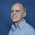## onsdag 9 oktober 2013

### Nobel Prize in Chemistry Awarded for Not Solving Schrödinger's Equation

Picture from presentation of Nobel Prize in Chemistry 2013: Multiscale Models

Quantum mechanics based on Schrödinger's equation as the core of modern physics, is presented as an almost perfect mathematical model of the atoms and molecules making up the world. But there is one hook: Schrödinger's equation can be solved analytically only for the Hydrogen atom with one electron and computationally only for atoms with few electrons; already Helium with two electrons poses difficulties.

The reason is that the Schrödinger equation is formulated using 3N spatial dimensions for an atom with N electrons with each electron demanding its own three-dimensional space. If each dimension takes 10 pixels to be resolved (very low accuracy), 10^100 = googol = 1 followed by 100 zeros pixels would be required for the Arsenic atom with 33 electrons, which is deadly poison for any thinkable computer, since the required number of pixels would be much larger than the number of atomic particles in the Universe!

Schrödinger's equation thus has to be replaced by an equation which is simpler to solve, and this is what computational chemistry is about. The first Nobel Prize in this field was given in 1998 to Kohn and Pople for so called density functional theory which reduces Schrödinger's equation to computable three spatial dimensions with say 100 pixels in each dimension. The next one was given today to Karplus, Lewitt and Warshel for
• the development of multiscale models for complex chemical systems:
• Karplus, Levitt and Warshel...managed to make Newton’s classical physics work side-by-side with the fundamentally different quantum physics.
• Previously, chemists had to choose to use either or. The strength of classical physics was that calculations were simple and could be used to model really large molecules.
• Its weakness, it offered no way to simulate chemical reactions. For that purpose, chemists instead had to use quantum physics.
• But such calculations required enormous computing power and could therefore only be carried out for small molecules.
• This year’s Nobel Laureates in chemistry took the best from both worlds and devised methods that use both classical and quantum physics.
• For instance, in simulations of how a drug couples to its target protein in the body, the computer performs quantum theoretical calculations on those atoms in the target protein that interact with the drug. The rest of the large protein is simulated using less demanding classical physics.
• Today the computer is just as important a tool for chemists as the test tube. Simulations are so realistic that they predict the outcome of traditional experiments.
These prizes were thus awarded for not solving the Schrödinger equation, while the very formulation of the equation (and the similar Dirac equation) was awarded in 1933.

In any case the prize was awarded to Computational Mathematics as an expression of The World as Computation.

#### 2 kommentarer:

1.I would call this mathematical (or calculational) heuristics, and it is NOT physical science. It is guessing, and guessing by computer at that. They might as well admit they are using fudge factors, and have done with the "progress in science" propaganda, because it is degeneration of science, not progression.

2.Well, a man can only what a man can do, and if solving the Schrödinger equation is beyond human capability, then you have to solve some other equation and that is not necessarily degeneration. It could be just realism, but it could also be fake science.